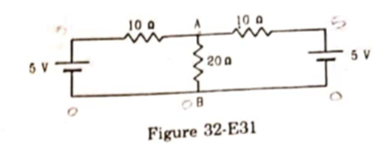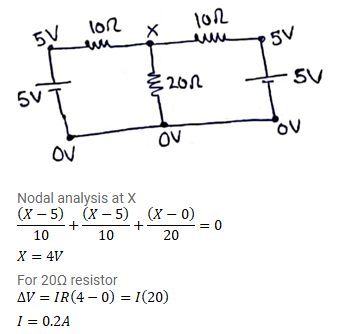# Find the current in the 20Ω resistor shown in figure (32-E31).

Question:

Find the current in the $20 \Omega$ resistor shown in figure (32-E31). If a capacitor of capacitance $4 \mu \mathrm{F}$ is joined between the points A and B, what would be the electrostatic energy stored in it in steady state.Solution: